# DAV Class 5 Maths Chapter 12 Worksheet 2 Solutions

The DAV Books Solutions Class 5 Maths and DAV Class 5 Maths Chapter 12 Worksheet 2 Solutions of Percentage offer comprehensive answers to textbook questions.

## DAV Class 5 Maths Ch 12 Worksheet 2 Solutions

Question 1.
Express the following as a fraction in the lowest term.
(a) 3%
Solution:
3% = $$\frac{3}{100}$$

(b) 35%
Solution:
35% = $$\frac{35}{100}$$ (Divide 35 and 100 by HCF 5)
= $$\frac{7}{20}$$

(c) 138%
Solution:
138% = $$\frac{138}{100}$$ (Divide 138 and 100 by HCF 2)
= $$\frac{69}{50}$$
= 1$$\frac{19}{50}$$(d) 675%
Solution:
675% = $$\frac{675}{100}$$ (Divide 675 and 100 by HCF 25)
= $$\frac{27}{4}$$
= 6$$\frac{3}{4}$$

(e) 80%
Solution:
80% = $$\frac{80}{100}$$ (Divide 80 and 100 by HCF 20)
= $$\frac{4}{5}$$

(f) 150%
Solution:
150% = $$\frac{150}{100}$$ (Divide 150 and 100 by HCF 50)
= $$\frac{3}{2}$$
= 1$$\frac{1}{2}$$

Question 2.
Express the following percentages into decimals.
(a) 0.2%
Solution:
The Decimal shifted by two places to the left when we converted percentages into decimals.
0.2% = $$\frac{0.2}{100}$$ = .002

(b) 5.6%
Solution:
The Decimal shifted by two places to the left when we converted percentages into decimals.
5.6% = $$\frac{5.6}{100}$$ = .056(c) 2.75%
Solution:
The Decimal shifted by two places to the left when we converted percentages into decimals.
2.75% = $$\frac{2.75}{100}$$ = .0275

(d) 3.8%
Solution:
The Decimal shifted by two places to the left when we converted percentages into decimals.
3.8% = $$\frac{3.8}{100}$$ = .038

(e) 1.9%
Solution:
The Decimal shifted by two places to the left when we converted percentages into decimals.
1.9% = $$\frac{1.9}{100}$$ = .019

(f) 9.5%
Solution:
The Decimal shifted by two places to the left when we converted percentages into decimals.
9.5% = $$\frac{9.5}{100}$$ = .095

Question 3.
Complete the table given below. The first is done for you.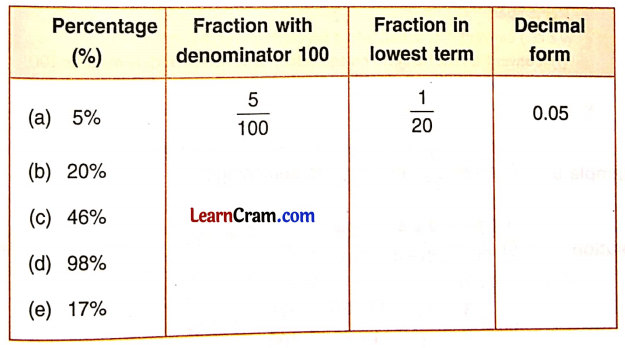Solution:Question 4.
Convert the following into fractions in the lowest term.
(a) $$\frac{3}{4}$$%
Solution: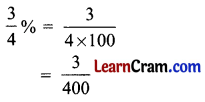(b) 16$$\frac{2}{3}$$%
Solution: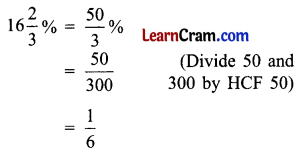(c) 33$$\frac{1}{3}$$%
Solution: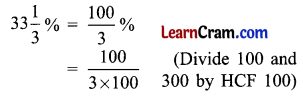$$\frac{1}{3}$$

(d) 7$$\frac{1}{7}$$%
Solution:(e) 11$$\frac{1}{4}$$%
Solution:(f) 12$$\frac{1}{2}$$%
Solution: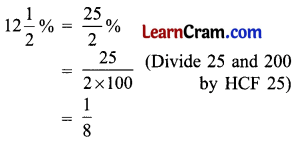DAV Class 5 Maths Chapter 12 Worksheet 2 Notes

Convert percentages into fractions in the lowest term.

Example 1.
Convert 25% into a fraction.
Solution:
25% = $$\frac{25}{100}$$ (Divide 25 & 100 100 by HCF 25)
= $$\frac{1}{4}$$

Convert percentages into decimal numbers.

Example 2.
Convert 35% into a decimal number.
Solution:
35% = $$\frac{35}{100}$$
= 0.35 (Decimal shifted by two places to the left)Example 3.
Convert 343% into a decimal number.
Solution:
343% = $$\frac{343}{100}$$
= 3.43 (Decimal shifted by two places to the left)